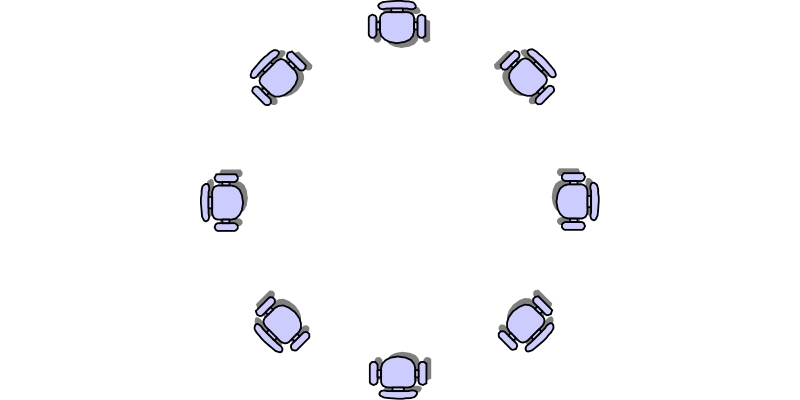### Seating Arrangement Reasoning Questions QuizSome conditions are given here. We have to follow those conditions and place the required person accordingly in a circular manner in around sitting arrangement, we can get question-based on.

#### 1. Instruction: Study the given information carefully and answer the following question. Eight-man P, Q, R, S, T, U, V, and W are sitting around a circular table facing the centre for having their lunch. V is not an immediate neighbour of R P is third to the right of R T is second to the left of R R is second to the left of Q V is second to the left of S P is second to the left of U Questions: Who is sitting to the immediate right of R?

 73880 V 73881 U 73882 S 73883 W

#### 2. Instruction: Study the given information carefully and answer the following question. Eight-man P, Q, R, S, T, U, V, and W are sitting around a circular table facing the centre for having their lunch. V is not an immediate neighbour of R P is third to the right of R T is second to the left of R R is second to the left of Q V is second to the left of S P is second to the left of U Questions: Who is sitting to the immediate left of W?

 73884 T 73885 V 73886 R 73887 P

#### 3. Instruction: Study the given information carefully and answer the following question. Eight-man P, Q, R, S, T, U, V, and W are sitting around a circular table facing the centre for having their lunch. V is not an immediate neighbour of R P is third to the right of R T is second to the left of R R is second to the left of Q V is second to the left of S P is second to the left of U Questions: Who is the following statement is true?

 73888 W is sitting between P and V 73889 W is to the immediate left of U 73890 D is sitting fifth to the left of W 73891 W is sitting between R and Q

#### 4. Instruction: Study the given information carefully and answer the following question. Eight-man P, Q, R, S, T, U, V, and W are sitting around a circular table facing the centre for having their lunch. V is not an immediate neighbour of R P is third to the right of R T is second to the left of R R is second to the left of Q V is second to the left of S P is second to the left of U Questions: Which of the following is the first person sitting between the second and third person?

 73892 PQV 73893 SRW 73894 RWQ 73895 UTS

#### 5. Instruction: Study the given information carefully and answer the following question. Eight-man P, Q, R, S, T, U, V, and W are sitting around a circular table facing the centre for having their lunch. V is not an immediate neighbour of R P is third to the right of R T is second to the left of R R is second to the left of Q V is second to the left of S P is second to the left of U Questions: Among the eight men which two men are not neighbour?

 73896 RW 73897 SP 73898 QP 73899 TU